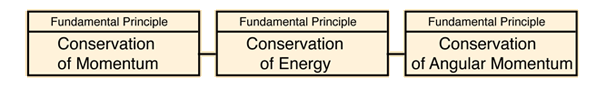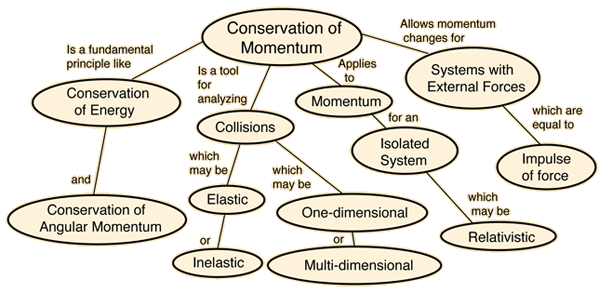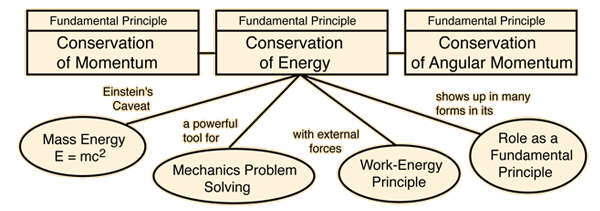# Conservation Laws

If a system does not interact with its environment in any way, then certain mechanical properties of the system cannot change. They are sometimes called "constants of the motion". These quantities are said to be "conserved" and the conservation laws which result can be considered to be the most fundamental principles of mechanics. In mechanics, examples of conserved quantities are energy, momentum, and angular momentum. The conservation laws are exact for an isolated system.Stated here as principles of mechanics, these conservation laws have far-reaching implications as symmetries of nature which we do not see violated. They serve as a strong constraint on any theory in any branch of science. There are other types of conservation laws that govern the behavior of nature in the quantum realm.

Index

 HyperPhysics***** Mechanics R Nave
Go Back

# Conservation of Momentum

The momentum of an isolated system is a constant. The vector sum of the momenta mv of all the objects of a system cannot be changed by interactions within the system. This puts a strong constraint on the types of motions which can occur in an isolated system. If one part of the system is given a momentum in a given direction, then some other part or parts of the system must simultaneously be given exactly the same momentum in the opposite direction. As far as we can tell, conservation of momentum is an absolute symmetry of nature. That is, we do not know of anything in nature that violates it.Index

 HyperPhysics***** Mechanics R Nave
Go Back

# Conservation of Energy

Energy can be defined as the capacity for doing work. It may exist in a variety of forms and may be transformed from one type of energy to another. However, these energy transformations are constrained by a fundamental principle, the Conservation of Energy principle. One way to state this principle is "Energy can neither be created nor destroyed". Another approach is to say that the total energy of an isolated system remains constant.Index

Energy concepts

 HyperPhysics***** Mechanics R Nave
Go Back

# Conservation of Energy as a Fundamental Principle

The conservation of energy principle is one of the foundation principles of all science disciplines. In varied areas of science there will be primary equations which can be seen to be just an appropriate reformulation of the principle of conservation of energy.

## First law of thermodyamics

Index

Energy concepts

 HyperPhysics***** Mechanics R Nave
Go Back

# Conservation of Angular Momentum

The angular momentum of an isolated system remains constant in both magnitude and direction. The angular momentum is defined as the product of the moment of inertia I and the angular velocity. The angular momentum is a vector quantity and the vector sum of the angular momenta of the parts of an isolated system is constant. This puts a strong constraint on the types of rotational motions which can occur in an isolated system. If one part of the system is given an angular momentum in a given direction, then some other part or parts of the system must simultaneously be given exactly the same angular momentum in the opposite direction. As far as we can tell, conservation of angular momentum is an absolute symmetry of nature. That is, we do not know of anything in nature that violates it.Rotating stool example
Index

 HyperPhysics***** Mechanics R Nave
Go Back

# An Isolated System

An isolated system implies a collection of matter which does not interact with the rest of the universe at all - and as far as we know there are really no such systems. There is no shield against gravity, and the electromagnetic force is infinite in range. But in order to focus on basic principles, it is useful to postulate such a system to clarify the nature of physical laws. In particular, the conservation laws can be presumed to be exact when referring to an isolated system:

Conservation of Energy: the total energy of the system is constant.
Conservation of Momentum: the mass times the velocity of the center of mass is constant.
Conservation of Angular Momentum: The total angular momentum of the system is constant.
Newton's Third Law: No net force can be generated within the system since all internal forces occur in opposing pairs. The acceleration of the center of mass is zero.

Index

 HyperPhysics***** Mechanics R Nave
Go Back